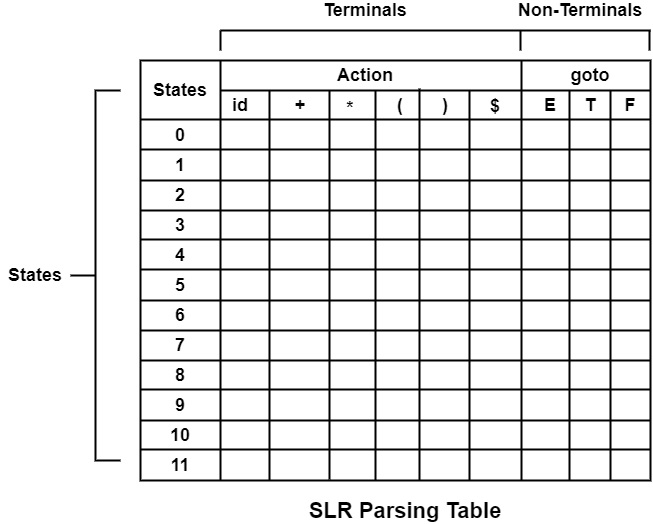# Construct SLR (1) parsing table for the grammar 1. E → E + T 2. E → T 3. T → T * F 4. T → F 5.F → (E) 6.F → id

Compiler DesignProgramming LanguagesComputer Programming

#### Web Design for Beginners: Build Websites in HTML & CSS 2022

68 Lectures 8 hours

#### HTML5 & CSS3 Site Design

61 Lectures 6 hours

Solution

Steps to produce SLR Parsing Table

• Generate Canonical set of LR (0) items

• Compute FOLLOW as required by Rule (2b) of Parsing Table Algorithm.

Computation of FOLLOW

By Rule (1) of FOLLOW

FOLLOW(E) = {$} (1) • E → E + T Applying Rule (2) FOLLOW i.e., comparing E → E + T with A → α B β  E → Ε E + T A → Α B Β ∴ A = E, α = ε, B = E, β = +T ∵ Since FIRST(β) = FIRST(+T) = {+}which does not contain ε. ∴ Rule (2b)of FOLLOW FOLLOW(E) = {+} (2) Applying Rule (3) of FOLLOW  E → Ε + T A → α B FOLLOW(T) = {FOLLOW(E)} (3) • E → T Rule (2) cannot be applied. As E → T cannot be compared with A → α B β. Applying Rule (3) of FOLLOW  E → ε T A → α B FOLLOW(T) = {FOLLOW(E)} (4) • T → T* F Applying Rule (2) of FOLLOW  T → E T *F A → A B β ∴ FIRST(β) = FIRST(∗ F) = {*} Rule (2a) ∴ FOLLOW (T) = {*} (5) Applying Rule (3) FOLLOW  T → T* F A → α B ∴ FOLLOW (F) = {FOLLOW(T)} (6) • T → F Rule (2) cannot be applied. As T → F cannot be compared with A → α B β Applying Rule (3)  T → ε F A → α B FOLLOW (F) = {FOLLOW(T)} (7) • F → (E) Applying Rule (2) FOLLOW  A → ( E ) F → A B β ∴ FIRST(β) = FIRST()) = { )} FOLLOW(E) = { )} (8) Rule (3) cannot be applied. • F → id Rule (2) and (3), both cannot be applied to this production. As they cannot be compared with F → id. Combining (1) to (8) FOLLOW(E) = {$}                                            (1)

FOLLOW(E) = {+}                                            (2)

FOLLOW(T) = {FOLLOW(E)}                          (3)

FOLLOW(T) = {FOLLOW(E)}                          (4)

FOLLOW (T) = {*}                                            (5)

FOLLOW (F) = {FOLLOW(T)}                          (6)

FOLLOW (F) = {FOLLOW(T)}                          (7)

FOLLOW(E) = { )}                                            (8)

∴ From (1), (2)and (8)

FOLLOW(E) = {$, +, )} From (3), (4), (5), (8) FOLLOW(T) = {$, +, ),*}

From (6) and (7)

FOLLOW(F) = {\$, +, ) *}

Construct the structure of a table in the following way −

• Write down all states I0 to I11(i. e. , 0 to 11) Row-wise.
• Write down the terminal symbols in Action column-wise.
• Write down the non-terminals column-wise in goto column-wise.